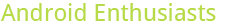## Maybe earned Yearling for this year

0

(click on this box to dismiss)Q&A about the site for enthusiasts and power users of the Android operating system

``````select year(v.CreationDate) Year,
count(distinct v.Id)*10 "Reputation gain"/*,
sum(iif(VoteTypeId = 1, 15, 0)) Accepted, -- Count both the asker and the answerer!
sum(iif(VoteTypeId = 2 and PostTypeId = 1, 10, 0)) "Question upvotes",
sum(iif(VoteTypeId = 3 and PostTypeId = 1, -2, 0)) "Question downvotes",
sum(iif(VoteTypeId = 3 and PostTypeId = 2, -3, 0)) "Answer downvotes", -- Don't forget the vote penalty!
sum(iif(VoteTypeId = 16, 2, 0)) Edits*/Hold tight while we fetch your results